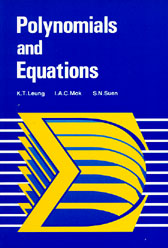{itemname} {itemname}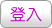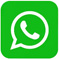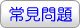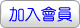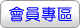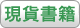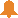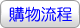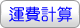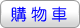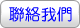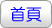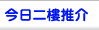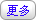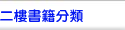Vectors, Matrices and Geometry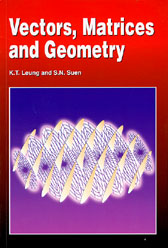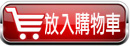沒有庫存 訂購需時10-14天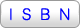9789622093607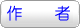K.T. Leung and S.N. Suen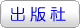香港大學出版社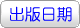1994年1月01日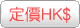95.00  元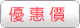HK\$ 85.5省下 \$9.5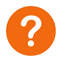[ 尚未分類 ]

This book is the last volume of a three-book series written for Sixth Form students and first-year undergraduates. It introduces the important concepts of finite-dimensional vector spaces through the careful study of Euclidean geometry. In turn, methods of linear algebra are then used in the study of coordinate transformations through which a complete classification of conic sections and quadric surfaces is obtained. The book concludes with a detailed treatment of linear equations in n variables in the language of vectors and matrices. Illustrative examples are included in the main text and numerous exercises are given in each section. The other books in the series are Fundamental Concepts of Mathematics (published 1988) and Polynomials and Equations (published 1992).

 其 他 著 作 1. Polynomials and Equations